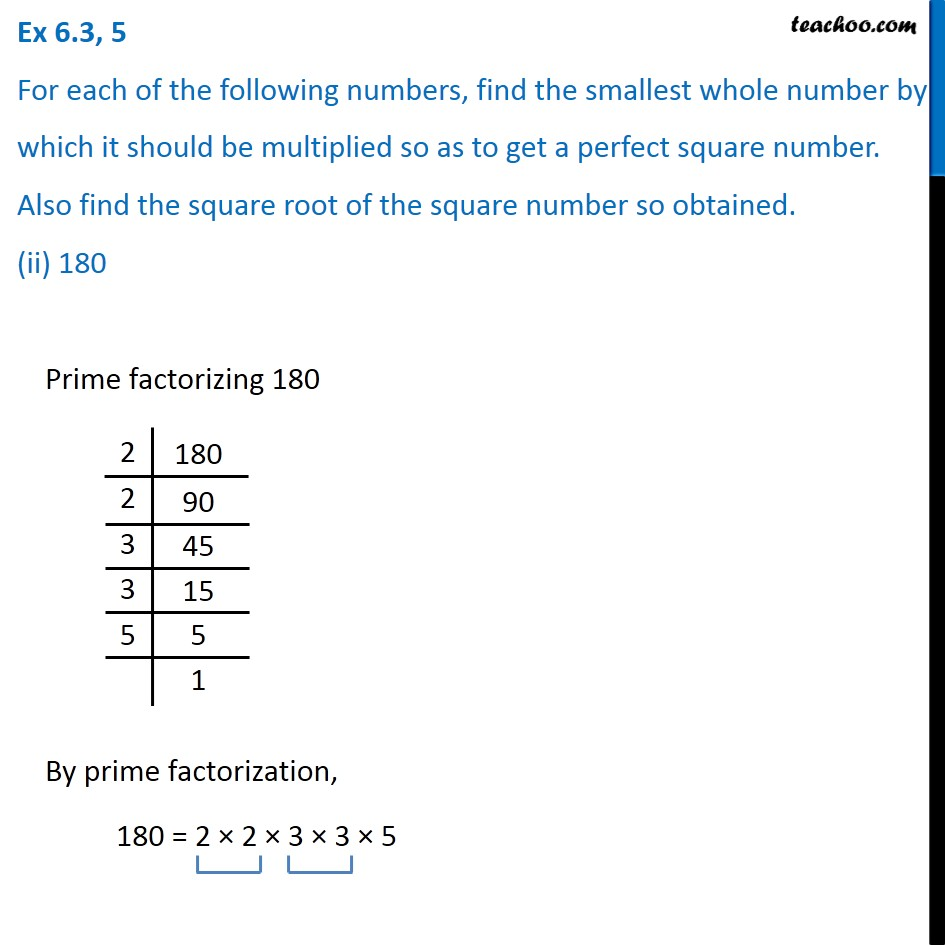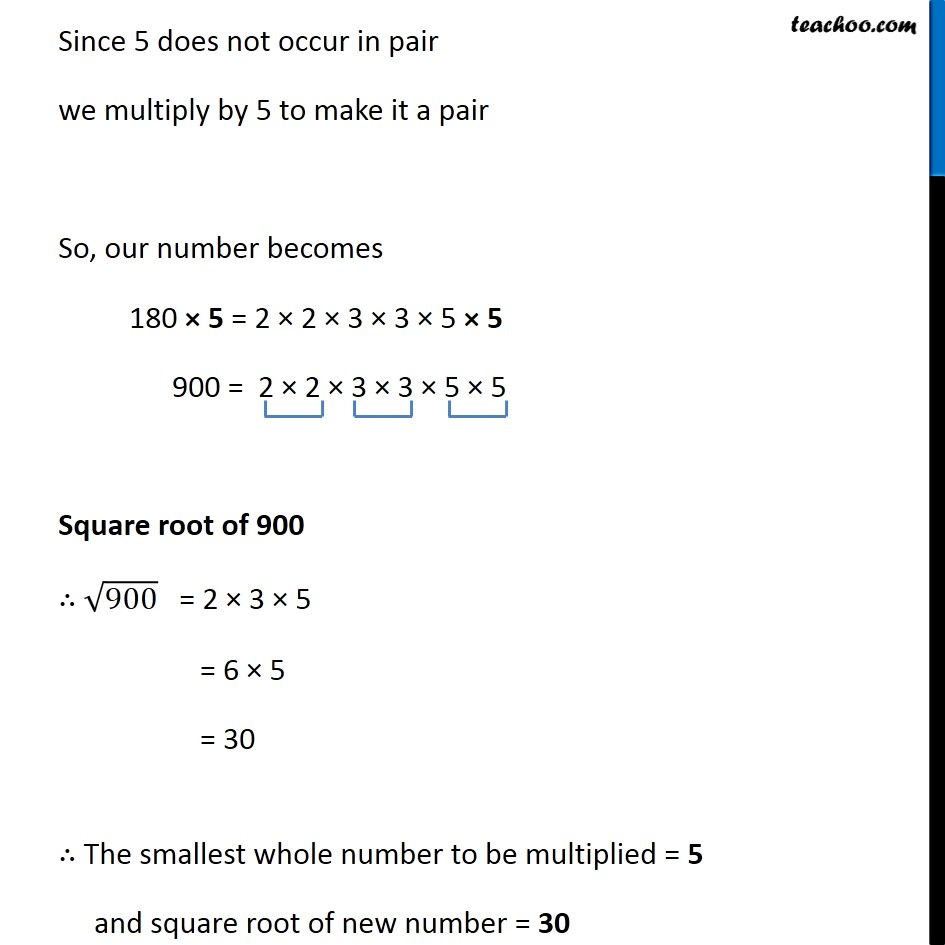1. Chapter 6 Class 8 Squares and Square Roots
2. Serial order wise
3. Ex 6.3

Transcript

Ex 6.3, 5 For each of the following numbers, find the smallest whole number by which it should be multiplied so as to get a perfect square number. Also find the square root of the square number so obtained. (ii) 180 Prime factorizing 180 By prime factorization, 180 = 2 × 2 × 3 × 3 × 5 Since 5 does not occur in pair we multiply by 5 to make it a pair So, our number becomes 180 × 5 = 2 × 2 × 3 × 3 × 5 × 5 900 = 2 × 2 × 3 × 3 × 5 × 5 Square root of 900 ∴ √900 = 2 × 3 × 5 = 6 × 5 = 30 ∴ The smallest whole number to be multiplied = 5 and square root of new number = 30

Ex 6.3

Chapter 6 Class 8 Squares and Square Roots
Serial order wise